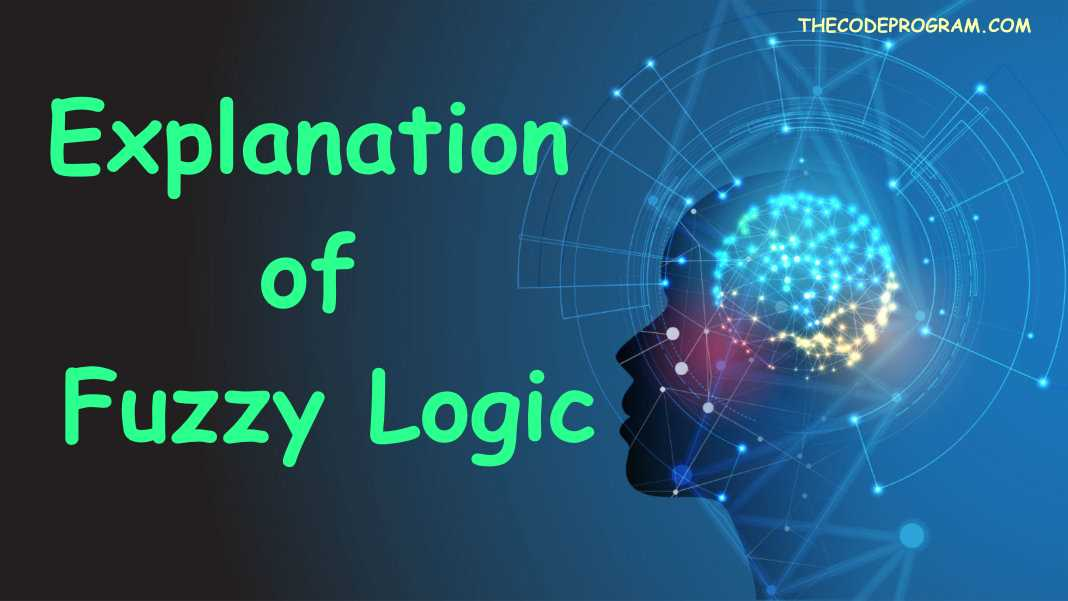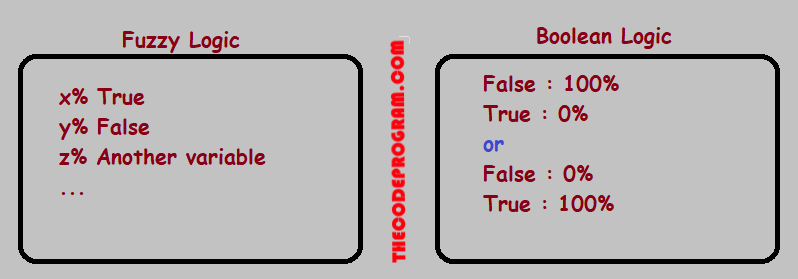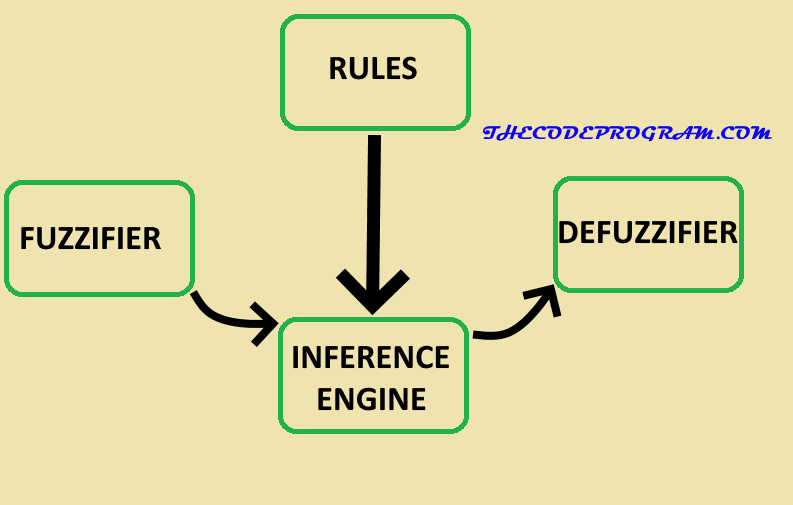## Explanation of Fuzzy Logic

Hello everyone, in this arricle we are going to talk about Fuzzy Logic and what can we do with the fuzzy logic and where can we use it.

Let's begin now.

### First What is Fuzzy?

The Fuzzy Term means not clear. In the real life actually nothing is clear and everything depend on a possibility. So we determine what is the result. And also the determining can be variable according to interpreter. So the is not the same for all interpreter. In here the situations specify by percentages. And generally we assume highest percentage value is the result.

We can compare the fuzzy logic with the Boolean logic. In boolean logic there is precision and everything is 100% true or 100% false. This means everything is clear. But in the fuzzY there is nothing clear. Fuzzy system is working with interpreting situtations and creating the percentages.

Below image you can see the relation between boolean logic and fuzzy logic.Why to use Fuzzy Logic?
• It helps the developer to understand human behaviour.
• It is greatly ideal for not clear processes.
• It is easy to implement to the machines.
• Can be used instead of Boolean logic when the choices more than two to determine.
• It will not probably give vertain results.
• If the fuzzy logic trained by an expert, the fuzzy logic can act like a human.

### Fuzzy Logic Architecture

Fuzzy logic has 4 parts to make its operations: These are:

• Fuzzifier
• Defuzzifier
• Rules
• Intelligence
Now lets explain them one by one:

Fuzzifier: This part of fuzzy logic measure the values from sensors or other type of inputs which is called as as Crisp Numbers. Thih part converts crisp numbers to the Fuzzy Sets. We can example them as humidity, temperature or something like them.

Defuzzifier: This part sets the output according to data produced by the inference engine and rules parts.

Rules: This part of fuzzy logic makes decisions of the system. All if/ else-if blocks are inside this part, and processed by this part of system. An expert should build this part to make good decisions.

Inference Engine: It helps you to determines the percentage of match between fuzzy input sets and the if/else rules. Based on the match, it specifies which rules need to be implemented according to the inputs.

Below Image you can see the Architecture of the fuzzy logic.Here some adventages of Fuzzy logic for the projects.

• The system can be make operatins and calculations with so many types of inputs and can create so many types of these related output.
• Fuzzy logic can be understanded easly
• With fuzzy logic the system can understand and operate the target devices effectively.

Some Applications of Fuzzy Logic:

• Fuzzy Logic is using in aviation industry to determine the very variable values like banking angles, Gust values at the tail.
• Using in the automotive systems to set the ideal speeding up, gearing up or braking.
• At he some companies to make quick answer systems.
• The advertising area for the best ad selection according to user web site visits.
• Fuzzy logic using for text and image processing

Have a great training the fuzzy system.

Burak Hamdi TUFAN.

19/04/2020

17/05/2020

16/07/2020